## Monday, October 31, 2016

### Azure Machine Learning: Classification Using Two-Class Averaged Perceptron

Today, we're going to walk through Sample 3: Cross Validation for Binary Classification Adult Dataset.  So far, the Azure ML samples have been interesting combinations of tools meant for learning the basics.  Now, we're finally going to get some actual Data Science!  To start, here's what the experiment looks like.Sample 3: Model Building and Cross-Validation
We had originally intended to skim over all of these models in a single post.  However, it was so interesting that we decided to break them out into separate posts.  So, we'll only be looking at the initial data import and the model on the far left, Two-Class Averaged Perceptron.  The first tool in this experiment is the Saved Dataset.Adult Census Income Binary Classification Dataset
As usual, the first step of any analysis is to bring in some data.  In this case, they chose to use a Saved Dataset called "Adult Census Income Binary Classification dataset".  This is one of the many sample datasets that's available in Azure ML Studio.  You can find it by navigating to Saved Datasets on the left side of the window.Sample Dataset
Let's take a peek at this data to see what we're dealing with.Adult Census Income Binary Classification Dataset (Visualize)
As you can see, this dataset has about 32k rows and 15 columns.  These columns appear to be descriptions of people.  We have some of the common demographic data, such as age, education, and occupation, with the addition of an income field at the end.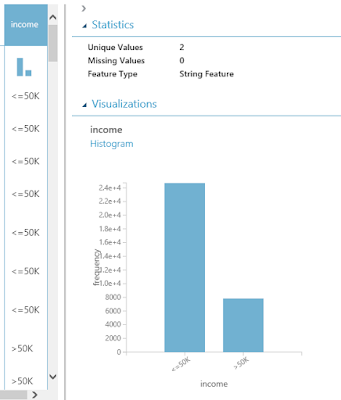Adult Census Income Binary Classification Dataset (Visualize) (Income)
This field takes two values, "<=50k" and ">50k", with the majority of people being in the lower bucket.  This would be a great dataset to do some predictive analytics on!  Let's move on to the next tool, Partition and Sample.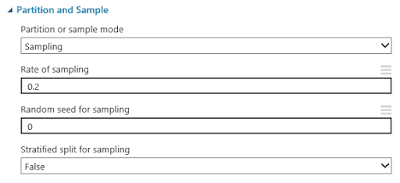Partition and Sample
This is a pretty cool tool that allows you to trim down your rows in a few different ways.  We could easily spend an entire post talking about this tool; so we'll keep it brief.  You have four different options for "Partition or Sample Mode".  In this sample, they have selected "Sampling" with a rate of .2 (or 20%).  This allows us to take random samples from our data.  We also have the "Head" option which allows us to pass through the top N rows, which would be really good if we were debugging a large experiment and didn't want to wait for the sampling algorithm to run.  We also have the option to sample Folds, which is another name for a partition or subset of data.  Let's take a quick look at the visualization to see if anything else is going on.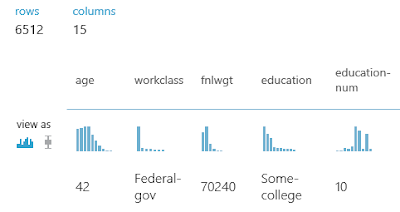Partition and Sample (Visualize)
We have the same 15 columns as before, the only difference is that we only 20% of the rows.  Nothing else seems be happening here.  Let's move on.Clean Missing Data
In a previous post, we've spoken about the Clean Missing Data tool in more detail.  To briefly summarize, you can tell Azure ML how to handle missing (or null) values.  In this case, we are telling the algorithm to replace missing values in all columns with 0.  Let's move on to the star of the show, Model Building.  We'll start with the model on the far left, Two-Class Averaged Perceptron.Two-Class Averaged Perceptron
This example is really interesting to us because we've never heard of it before writing this.  The Two-Class Averaged Perceptron algorithm is actually quite simple.  It takes a large number of numeric variables (it will automatically translate Categorical data into Numeric if you give it any.  These new variables are called Dummy Variables.).  Then, it multiplies the input variables by weights and adds them together to produce a numeric output.  That output is a score that can be used to choose between two different classes.  In our case, these classes are "Income <= 50k" and "Income > 50k".  Some of you might think this logic sounds very similar to a Neural Network.  In fact, the Two-Class Averaged Perceptron algorithm is a simple implementation of a Neural Network.

This algorithm gives us the option of providing three main parameters, "Create Trainer Mode", "Learning Rate" and "Maximum Number of Iterations".  "Learning Rate" determines how many steps the algorithm takes in order to calculate the "best" set of weights.  If the "Learning Rate" is too high (making the number of steps too low), the model will train very quickly, but the weights may not be a very good fit.  If the "Learning Rate" is too low (making the number of steps too high), the model will train very slowly, but could possibly produce "better" weights.  There are also concerns of Overfitting and Local Extrema to contend with.

"Maximum Number of Iterations" determines how many times times the model is trained.  Since this is an Averaged Perceptron algorithm, you can run the algorithm more than once.  This will allow the algorithm to develop a number of different sets of weights (10 in our case).  These sets of weights can be averaged together to get a final set of weights, which can then be used to classify new values.  In practice, we could achieve the same result by creating 10 scores using the 10 sets of weights, then averaging the scores.  However, that method would seem to be far less efficient.

Finally, we have the "Create Trainer Mode" parameter.  This parameter allows us to pass in a single set of parameters (which is what we are currently doing) or pass in multiple sets of parameters.  You can find more information about this algorithm here and here.

This leaves us with a few questions that perhaps some readers could help us out with.  If you have 10 iterations, but set a specific random seed, does it create the same model 10 times, then average 10 identical weight vectors to get a single weight vector?  Does it use the random seed to create 10 new random seeds, which are then used to create 10 different weight vectors?  What happens if you define a set of 3 Learning Rates and 10 Iterations?  Will the algorithm run 30 iterations or will it break the iterations into sets of 3, 3, and 4 to accomodate each of the learning rates?  If you know the answers to these questions, please let us know in the comments.  Out of curiosity, let's see what's under Visualize for this tool.Two-Class Averaged Perceptron (Visualize)
This is interesting.  These aren't the same parameters that we input into the tool, nor do they seem to be affected by our data stream.  This is a great opportunity to point out the way that models are built in Azure ML.  Let's take a look at data flowData Flow
I've rearranged the tools slightly to make it more obvious.  As you can see, the data does not flow into the model directly.  Instead, the model metadata is built using the model tool (Two-Class Averaged Perceptron in this class).  Then, the model metadata and the sample data are consumed by whatever tool we want to use downstream (Cross Validate Model in this case).  This means that we can reuse a model multiple times just by attaching it to different branches of a data stream.  This is especially useful when we want to use the same model against different data sets.  Let's move on to the final tool in this experiment, Cross Validate Model.Cross Validate Model
Cross-Validation is a technique for testing, or "validating", a model.  Most people would test a model by using a Testing/Training split.  This means that we split our data into two separate sets, one for training the model and another one for testing the model.  This methodology is great because it allows us to test our model using data that it has never seen before.  However, this method is very susceptible to bias if we don't sample our data properly, as well as sample size issues.  This is where Cross-Validation comes in.

Imagine that we used the Testing/Training method to create a model using the Training data, then tested the model using the Testing data.  We could estimate how accurate our model is by seeing how well it predicts known values from our Testing data.  But, how do we know that we didn't get lucky?  How do we know there isn't some strange relationship in our data that caused our model to predict our Testing data well, but predict real-world data poorly?  To do this, we would want to train the model multiple times using multiple sets of data.  So, we separate our data into 10 sets.  Nine of the sets are used to train the model, and the remaining set is used to test.  We could repeat this process nine more times by changing which of the sets we use to test.  This would mean that we have created 10 separate models using 10 different training sets, and used them to predict 10 mutually exclusive testing sets.  This is Cross-Validation.  You can find out more about Cross-Validation here.  Let's see it in action.Scored Results (1)
There are two outputs from the Cross Validate Model tool, Scored Results and Evaluation Results by Fold.  The Scored Results output shows us the same data that we passed into the tool, with 3 additional columns.  The first column, Fold Assignments, is added to the start of the data.  This tells us which of the 10 sets, or "folds", the row was sampled into.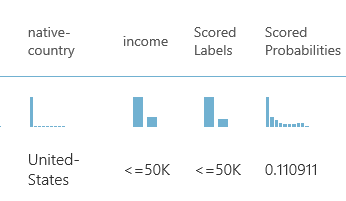Scored Results (2)
The remaining columns, Scored Labels and Scored Probabilities, are added to the end of the data.  The Scored Labels column tells us which category the model predicted this row would fall into.  This is what we were looking for all along.  The Scored Probability is a bit more complicated.  Mathematically, the algorithm wasn't trying to predict whether Income was "<=50k" or ">50k".  It was only trying to predict ">50k" because in a Two-Class algorithm, if you aren't ">50k", then you must be "<=50k".  If you looked down the Scored Probabilities column, you would see that all Scored Probabilities less than .5 have a Scored Label of "<=50k" and all Scored Probabilities greater than .5 have a Scored Label of ">50k".  If were using a Multi-Class algorithm, it would be far more complicated.  If you want to learn about the Two-Class Average Perceptron algorithm, read here and here.

There is one neat thing we wanted to show using this visualization though.Scored Results (Comparison)
When we click on the "Income" column, a histogram will pop up on the right side of the window.  If we click on the "Compare To" drop-down, and select "Scored Labels", we get a very interesting chart.Contingency Table
This is called a Contingency Table, also known as a Confusion Matrix or a Crosstab.  It shows you the distribution of your correct and incorrect predictions.  As you can see, our model is very good at predicting when a person has an Income of "<=50k", but not very good at predicting ">50k".  We could go much deeper into the concept of model validation, but this was an interesting chart that we stumbled upon here.  Let's look at the Evaluation Results by Fold.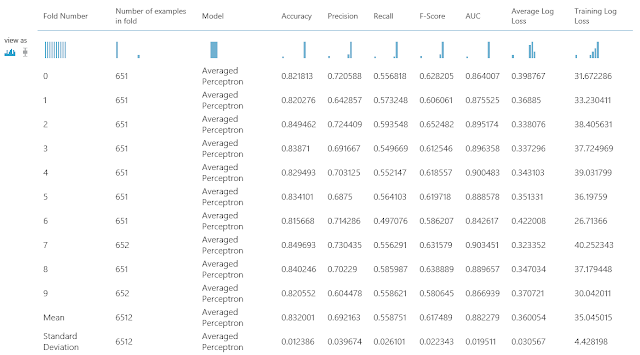Evaluation Results by Fold
This shows you a bunch of statistics about your Cross-Validation.  The purpose of this post was to talk about the Two-Class Averaged Perceptron, so we won't spend much time here.  However, don't be surprised if we make a full-length post about this in the future because there is a lot of information here.

We hope that this post sparked as much excitement in you as it did in us.  We're really starting to see just how much awesomeness is packed into Azure Machine Learning Studio; and we're so excited to keep digging.  Thanks for reading.  We hope you found this informative.

BI Engineer
Valorem Consulting
@BreakingBI
llewellyn.wb@gmail.com

## Monday, October 10, 2016

### Azure Machine Learning: Edit Metadata, Clean Missing Data, Evaluate Probability Function, Select Columns and Compute Linear Correlation

Today, we're going to look at Sample 2: Dataset Processing and Analysis: Auto Imports Regression Dataset in Azure ML.  In our previous post, we took our first look inside Azure ML using a simple example.  Now, we're going to take it a step further with a larger experiment.  Let's start by looking at the whole experiment.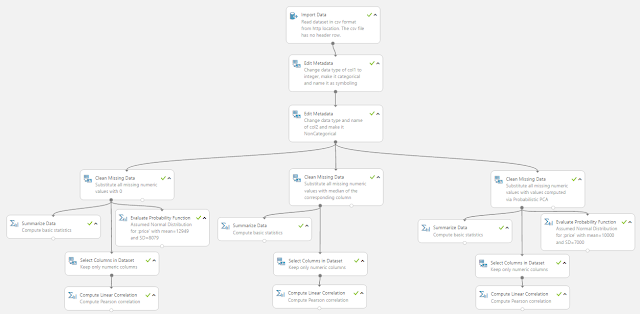Sample 2
This definitely looks daunting at first glance.  So, let's break it down into smaller pieces.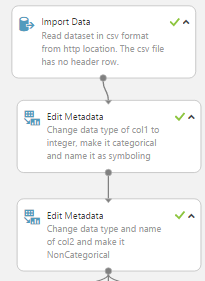Data Import
As you can see, this phase is made up of an HTTP Data Import, which we covered in the previous post.  However, this Edit Metadata tool is interesting.  Let's take a look inside.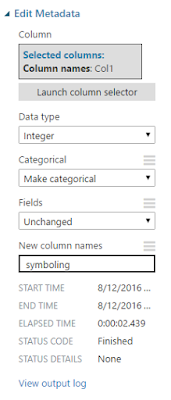Edit Metadata
In most tools, you don't "replace" columns by editing metadata.  For instance, if you were using a data manipulation tool like SSIS, you would have to use one tool to create the new columns with the new data types, then use another tool to remove the old columns.  This is not only cumbersome from a coding perspective, but it's also performs inefficiently because you have to carry those old columns to another tool after you no longer need them.

The Edit Metadata tool on the other hand, does both of these in one.  It allows you rename your columns, change data types, and even change them from categorical to non-categorical.  There's also a long-list of options in the "Fields" box to choose from.  We're not sure what any of these options do, but that sounds like a great topic for another post!  Alas, we're veering off-topic.

One of the major things we don't like about this tool is that it sets one set of changes for the entire list of columns.  That means that if you want multiple sets of changes, you need to use the tool multiple times.  Fortunately, this experiment only uses it twice.  Before we move on, let's take a look at the data coming out of the second Edit Metadata tool.Data Before Analysis
The two edit metadata tools altered columns 1, 2 and 26, giving them names and making them numeric.  This leads us with one huge question.  Why did they not rename the rest of the columns?  Do they not intend on using them later or were they just showcasing functionality?  Guess we'll just have to find out.

Let's move on to the left set of tools.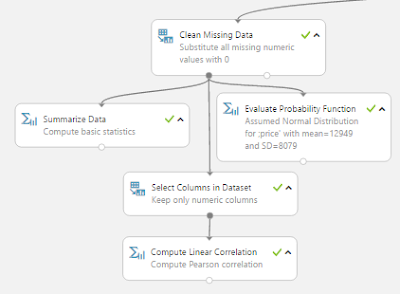Left Set
This set starts off with a Clean Missing Data tool.  Let's see what it does.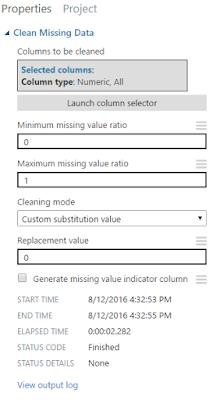Clean Missing Data (Left)
As you can see, this tool takes all numeric columns, and substitutes 0 any time that the value is missing.  The process of replacing missing values is called Imputation and it's a big deal in the data science world.  You can read up on it here.  This tool also has the option to generate a missing value indicator column.  This means that any row where a value was imputed would a value of 1 (or TRUE) and all other rows would have a value of 0 in the column.  While we would love to go in-depth about imputation, it's a subject we'll have to reserve for a later post.

In our opinion, the coolest part about this tool has nothing to do with its goal.  It has one of the best column selection interfaces we've ever seen.  It allows you to programatically add or remove columns from your dataset. Looking at some of the other tools, this column selection interface pops in up in quite a few of them.  This makes us very happy.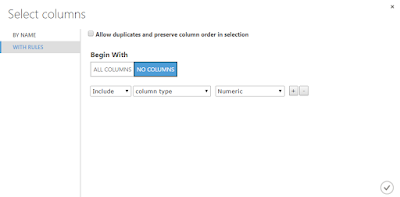Select Columns
We'll skip over the Summarize Data tool, as that was covered in the previous post.  Let's move on to the Evaluate Probability Function tool.Evaluate Probability Function (Left)
There are a couple of things to note about this tool.  First, it lets you pick from a very large list of distributions.Distributions
It chose to use the Normal Distribution, which is what people are talking about when they say "Bell-Shaped Curve".  It's definitely the most common used algorithm by beginner data scientists.  Next, it lets you select which method you would like to use.Method
There are three primary methods in statistics, the Probability Density Function (PDF), Cumulative Distribution Function (CDF), and Inverse Cumulative Distribution Function (Inverse CDF).  If you want to look these up on your own, you can use the following links: PDF, CDF, InverseCDF.

You can get entire degrees just by learning these three concepts and we won't even attempt to explain them in a sentence.  Simply put, if you want to see how likely (or unlikely) an observation is, use the CDF.  We'll leave the other two for you to research on your own.  Let's move on to the Select Columns tool.Select Columns (Left)
This tool is about as simple as it comes.  You tell it which columns you want (using that awesome column selector) and it throws the rest away.  Let's move on to the final tool, Compute Linear Correlation.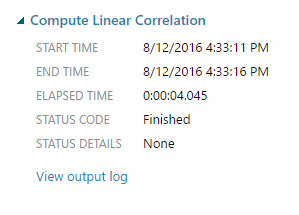Compute Linear Correlation (Left)
This is another simple tool.  You give it a data set with a bunch of numeric columns, it spits out a matrix of Pearson correlations.Linear (Pearson) Correlations (Left)
These are the traditional correlations you learned back in high school.  A correlation of 0 means that there is no linear relationship and a correlation of 1 or -1 means there is a perfect linear correlation.

We were planning on moving on to the other legs of this experiment.  However, they are just slightly altered versions of what we went through.  This is an interesting sample for Microsoft to publish, as it doesn't seem to have any real analytical value.  We can't imagine using this is any sort of investigative or analytic scenario.  What is it good for then?  Learning Azure ML!  This is exactly what we used it for.  It showed us some neat features and prompted a few good follow-up posts to get more in-depth on some of these tools.  Hopefully, it sparked some good ideas in you guys too.  Thanks for reading.  We hope you found this informative.